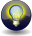#Boost C++ Libraries

...one of the most highly regarded and expertly designed C++ library projects in the world.

#### More About Attributes of Compound Components

While parsing input or generating output it is often desirable to combine some constant elements with variable parts. For instance, let us look at the example of parsing or formatting a complex number, which is written as `(real, imag)`, where `real` and `imag ` are the variables representing the real and imaginary parts of our complex number. This can be achieved by writing:

Library

Sequence expression

Qi

```'(' >> double_ >> ", " >> double_ >> ')'```

Karma

```'(' << double_ << ", " << double_ << ')'```

Fortunately, literals (such as `'('` and `", "`) do not expose any attribute (well actually, they do expose the special type `unused_type`, but in this context `unused_type` is interpreted as if the component does not expose any attribute at all). It is very important to understand that the literals don't consume any of the elements of a fusion sequence passed to this component sequence. As said, they just don't expose any attribute and don't produce (consume) any data. The following example shows this:

```// the following parses "(1.0, 2.0)" into a pair of double
std::string input("(1.0, 2.0)");
std::string::iterator strbegin = input.begin();
std::pair<double, double> p;
qi::parse(strbegin, input.end(),
'(' >> qi::double_ >> ", " >> qi::double_ >> ')', // parser grammar
p);                                               // attribute to fill while parsing
```

and here is the equivalent Spirit.Karma code snippet:

```// the following generates: (1.0, 2.0)
std::string str;
std::back_insert_iterator<std::string> out(str);
generate(out,
'(' << karma::double_ << ", " << karma::double_ << ')', // generator grammar (format description)
p);                                                     // data to use as the attribute
```

where the first element of the pair passed in as the data to generate is still associated with the first `double_`, and the second element is associated with the second `double_` generator.

This behavior should be familiar as it conforms to the way other input and output formatting libraries such as `scanf`, `printf` or `boost::format` are handling their variable parts. In this context you can think about Spirit.Qi's and Spirit.Karma's primitive components (such as the `double_` above) as of being type safe placeholders for the attribute values.

TipSimilarly to the tip provided above, this example could be rewritten using Spirit's multi-attribute API function: ```double d1 = 0.0, d2 = 0.0; qi::parse(begin, end, '(' >> qi::double_ >> ", " >> qi::double_ >> ')', d1, d2); karma::generate(out, '(' << karma::double_ << ", " << karma::double_ << ')', d1, d2); ``` which provides a clear and comfortable syntax, more similar to the placeholder based syntax as exposed by `printf` or `boost::format`.

Let's take a look at this from a more formal perspective. The sequence attribute propagation rules define a special behavior if generators exposing `unused_type` as their attribute are involved (see Generator Compound Attribute Rules):

Library

Sequence attribute propagation rule

Qi

```a: A, b: Unused --> (a >> b): A```

Karma

```a: A, b: Unused --> (a << b): A```

Given `a` and `b` are parsers (generators), and `A` is the attribute type of `a`, and `unused_type` is the attribute type of `b`, then the attribute type of ```a >> b``` (```a << b```) will be `A` as well. This rule applies regardless of the position the element exposing the `unused_type` is at.
This rule is the key to the understanding of the attribute handling in sequences as soon as literals are involved. It is as if elements with `unused_type` attributes 'disappeared' during attribute propagation. Notably, this is not only true for sequences but for any compound components. For instance, for alternative components the corresponding rule is:
```a: A, b: Unused --> (a | b): A